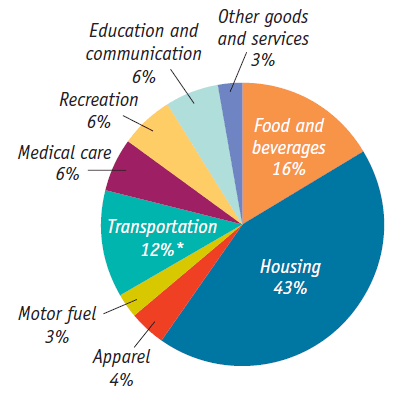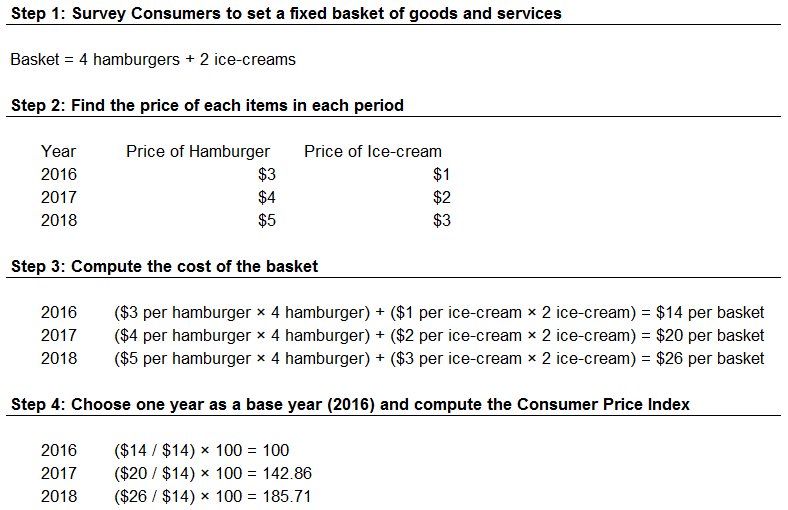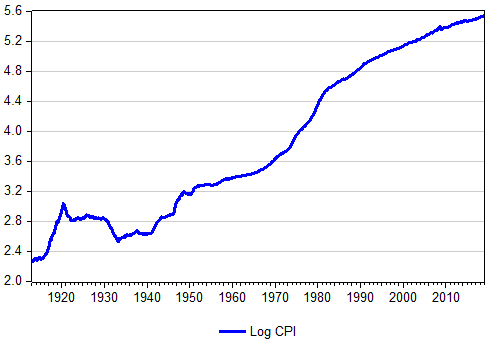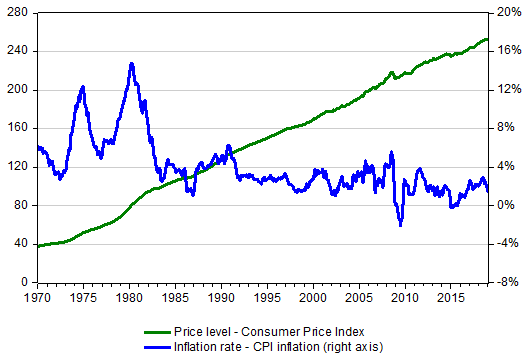# CPI Inflation Calculator (Consumer Price Index)

Created by Tibor Pal, PhD candidate and Dariusz Walczy-Antkowicz
Reviewed by Bogna Szyk and Jack Bowater
Last updated: Feb 10, 2021

CPI Inflation Calculator is a tool to estimate the change in the general level of prices in the United States over different time intervals. It relies on the Consumer Price Index data, which is published by the U.S. Bureau of Labor Statistics (BLS CPI) on a monthly basis. If you read on, you will learn what is the Consumer Price Index (CPI) and the CPI formula, so you will know how to calculate CPI and CPI inflation. Besides, we will elaborate on some important aspects of CPI in economics.

## What is consumer price index (CPI) - the CPI definition

There are several ways to measure how much certain goods cost in a given year. Still, the most broadly applied measure of the overall price level in the United States is the Consumer Price Index (CPI). CPI was designed to reveal how the cost of all purchases by a typical American household has changed over time (the other popular measures are the GDP deflator or the Producer Price Index).

CPI is computed by surveying market prices for a particular basket of goods and services that is devised to represent the consumption of a typical family of four living in a typical American city.

The market basket used to compute the CPI is an intricate amalgam of goods and services that aims to reflect the actual spending patterns for the U.S. population. To obtain the necessary data, the Bureau of Labor Statistics (BLS) delegates its employees to about 23,000 retail outlets in 87 cities. Every month it registers around 80,000 prices covering a broad range of items that are converted into a single index measuring the overall level of prices.

## How to calculate CPI - the CPI formula

To give you some insight on how statisticians estimate this compact single number, let's reduce the range of purchases of a typical family to only two goods: hamburgers and ice-creams. The following four steps represent the main stages of the computation of the CPI index.

The goods and services included in the basket need to correspond to the real need of a typical consumer. If a product are more important than another, its price needs to be assigned a higher weight. In other words, the basket should be aimed to reflect the general cost of living. For example, if a typical family consumes more hamburgers than ice-cream, the price of a hamburger needs to be included with a higher quantity. In our case, an average household buys 4 burgers and 2 ice-creams.

For the sake of reality, the below graph represents the percentage shares of the main types of spending in the US CPI as of December 2008.Source: Ray, M. and Anderson, D. - Krugman's Economics for AP - 2011 - Worth Publishers

2. Determining prices

Prices of the items included in the basket need to be recorded regularly. In our example, the prices of hamburgers and ice-creams are stated in three different years.

The number of items needs to be multiplied by its price for each period.

4. Computing the index

There must be a base year, designated arbitrarily, that represents a benchmark period (the benchmark index of BLS CPI is the average value between 1982 and 1984). Then finally we arrive at the point when the Consumer Price Index can be computed by the following CPI equation:

Consumer price index = Price of a basket of goods and services in the current period / Price of the basket in the base period * 100.

The below table summarizes the CPI calculation procedure for our example.## What is CPI Inflation - how to calculate CPI inflation rate

The inflation rate is one of the most important indicators in economics. As it was mentioned, it is the percentage change in the general price level over a period of time. Typically it covers a year, however, with our calculator, you can define the US CPI inflation rate in any arbitrary interval starting from 1913.

The formula applied here is the following:

CPI inflation rate = (CPI in target year - CPI in reference year) / CPI in reference year * 100

Turning back to our previous example, we can compute the yearly CPI inflation rate for our hypothetical basket in 2017 and 2018.

CPI inflation rate in 2017 = (142.86 - 100.00) / 100.00 * 100 = 42.86 %

CPI inflation rate in 2018 = (185.71 - 142.8) / 142.8 * 100 = 30.05 %

The altering trend in the inflation rate between 1913 and 2018 can be tracked easily on the graph below, where the CPI is represented on a logarithmic scale. In this way, the slope of the curve indicates the speed of change in the CPI.Since the 1940s, the CPI has risen continually; however, its annual percent changes in the last 30 years have been much smaller than those of the 1970s and early 1980s.

## How to use the CPI inflation calculator

You can use this calculator to compute the yearly inflation rate, but you can also estimate the cumulative inflation rate for any arbitrary interval from 1913 to today. Besides, you can learn the average yearly inflation rate (by applying the Compound Annual Growth Rate formula) in the chosen range.

The first step is to fix the interval by setting two dates: the reference year, that represent the year from the computation is applied, and the target year, that is the last year taken into account.

For example, if you are curious about what was the cumulative CPI inflation rate during a hundred years between 1918 and 2018, you need to set the years accordingly:

Reference year = 1918

Target year = 2018

Then you receive the results immediately based on the BLS CPI data also showed in the platform.

CPI inflation rate = (CPI 2018 - CPI 1918) / CPI 1918 * 100 = (251.1 - 15.1) / 15.1 * 100 = 1,563 %

Average CPI inflation rate = ((CPI 2018 / CPI 1918) ^ (1 / number of years ) - 1) * 100 = ((251.1 / 15.1) ^ (1 / 100) - 1 ) * 100 = 2.85 %

Thus, the cumulative CPI inflation rate and the average yearly CPI inflation rate from 1918 to 2018 was 1,563 % and 2.85% respectively.

## Consumer Price Index (CPI) vs. CPI inflation

As the name of the calculator suggests, here we deal prominently with the CPI inflation rate. Yet to understand its background we need to take a slight departure and visit the Consumer Price Index as well.

Before we go into details, it is of great importance to distinguish between the level of prices and the inflation rate. Although the two phrases are strongly connected, their economic implications are very different.

As a starting point, one way to demonstrate the difference between the two indicators is to display them on a graph. On the below illustration CPI represents the level of prices and CPI inflation embodies the annual inflation rate.As you can see, over the past 50 years, the price level has been following an upward tendency (the steady increase was interrupted only in 2009 after the onset of the Global Financial Crisis). However the inflation rate - the rate at which consumer prices change - has been following a different course.

The CPI shows that the price level in the United States in 2019 is more than 6 times higher compared to prices in 1970 (thus the buying power of dollar has fallen considerably) but how did the substantial increase affect the overall economy?

To get the answer, we need to take into consideration not only the level of prices but also the level of income: what really matters is the real wage - the nominal wage divided by the price level. In other words, we should rather be concerned about the range of goods and services that we can afford from our salaries: if wages increase at the same rate as prices do, we are in the same position regardless of their nominal values.

It follows that the massive surge in prices that has occurred since the early 1970s hasn't made people in the United States poorer: as incomes were raised by a higher rate, real income has become even higher.

Now as we have some insight on the overall level of prices, let's focus on its yearly changes, that is, the CPI inflation rate.

You may think if the price level alone doesn't really matter, the rate of its change doesn't either. Let's briefly scrutinize the US CPI inflation rate in the last 50 years. In the 70s, the US economy was struggling with a relatively high inflation rate that moderated from the 90s. However, as a result of the 2008 financial turmoil and the following recession (a negative GDP growth at least in two consecutive quarters), the inflation rate in several periods became negative (inflation rate below zero percent corresponds to a decrease in the general price level of goods and services that is called deflation).

There is a broad consensus among economists that the rapid rise in the overall price level harms the economy as it implies substantial economic costs. These costs, in general, fall into three main groups:

• Shoe-Leather Costs

The high inflation rate is associated with a fast decline in the buying power of the given currency. As a result, people tend to eschew holding that currency and instead proceed transactions that often imply a considerable economic cost (for example a frequent and broad exchange of money into a stable currency might require a sizable workload in the banking sector that deprives real resources of an economy).

The expression of "shoe-leather costs" refers to the wear and tear caused by the extra running around that happens when people are trying to avoid holding money. This type of cost is relatively small in moderately high inflation, it becomes destructive at the time of hyperinflation.

In our world, most of the things that you can find in a shop have a listed price. Updating this price has a real cost, called the menu cost. The frequent updates of prices can amplify the adverse effect of menu cost on the economy: the higher the inflation rate, the higher the menu cost. Though, with technological improvements, menu costs are becoming less and less relevant, since prices can be updated electronically, which requires less workload.

• Unit-of-Account Costs

We make economic decisions and conduct our businesses based on the value of the dollar (or any other specific currency). In other words, the value of material things (for example goods, services, labor, income, expenses, profits, liability, debt or savings) are measured and compared in a specific currency. This function of the dollar is called the unit-of-account. Inflation weakens such a feature of the currency, as the rapid decline of the purchasing power of a dollar imposes uncertainty and reduce the quality of economic decisions.

To sum up, what really matters for the economy as a whole is not the overall price level but the inflation rate, the rate how the general price level changes.

On the other hand, deflation is not a welcomed phenomenon neither. It may not only delay consumption and investment decisions, but it might increase the real debt burden of loans thus further worsening the financial position of people in debt. Deflation is particularly harmful when a considerable part of the households are indebted, for example, as it happened after the 2008 financial crisis. In this situation, the real values of loans increase, further hindering the economic recovery.

• Mankiw, G. - Macroeconomics (8th edition) - 2012 - Worth Publishers

• Ray, M. and Anderson, D. - Krugman's Economics for AP - 2011 - Worth Publishers

Tibor Pal, PhD candidate and Dariusz Walczy-Antkowicz
Reference year
Annual CPI in the reference year
Target year
Annual CPI in the target year
270.97
Cumulative rate of CPI inflation
%
Average CPI inflation rate
%
People also viewed…

### Books vs e-Books